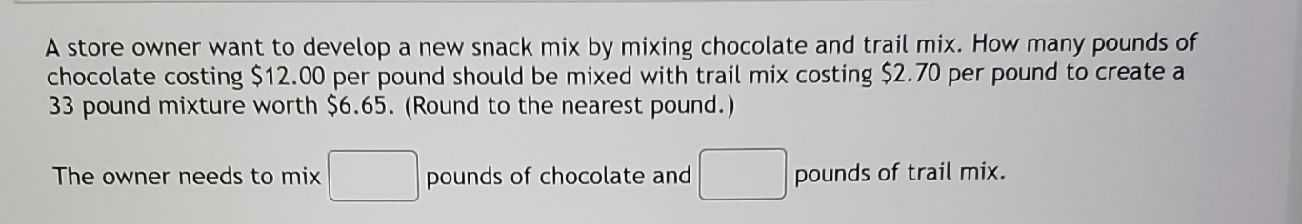### Still have math questions?

Algebra
QuestionA store owner want to develop a new snack mix by mixing chocolate and trail mix. How many pounds of chocolate costing $$\ 12.00$$ per pound should be mixed with trail mix costing $$\ 2.70$$ per pound to create a $$33$$ pound mixture worth $$\ 6.65 .$$ (Round to the nearest pound.)

The owner needs to mix $$\square$$ pounds of chocolate and $$\square$$ pounds of trail mix.